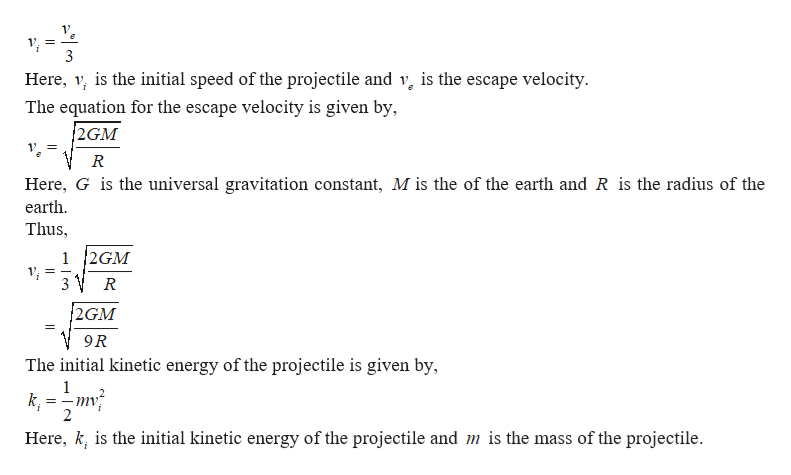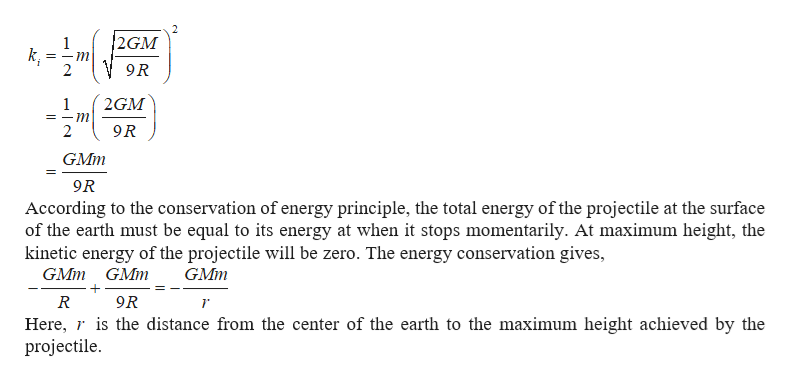# A projectile is fired straight upward from the Earth’s surfaceat the South Pole with an initial speed equal to one third theescape speed. (a) Ignoring air resistance, determine how farfrom the center of the Earth the projectile travels before stoppingmomentarily. (b) What is the altitude of the projectile atthis instant?

Question
11 views

A projectile is fired straight upward from the Earth’s surface
at the South Pole with an initial speed equal to one third the
escape speed. (a) Ignoring air resistance, determine how far
from the center of the Earth the projectile travels before stopping
momentarily. (b) What is the altitude of the projectile at
this instant?

check_circle

Step 1

(a)The initial speed of the projectile is given by,help_outlineImage Transcriptionclose3 Here, v, is the initial speed of the projectile and v, is the escape velocity. The equation for the escape velocity is given by, 2GM Ve Here, G is the universal gravitation constant, M is the of the earth and R is the radius of the earth. Thus, 1 2GM V; = 2GM 9R The initial kinetic energy of the projectile is given by, k, 2 kinetic energy of the projectile and m is the mass of the projectile. Here, k, is the init fullscreen
Step 2

Thus,

...help_outlineImage Transcriptionclose2GM 1 k, 9R 2GM 9R GMm 9R According to the conservation of energy principle, the total energy of the projectile at the surface of the earth must be equal to its energy at when it stops momentarily. At maximum height, the kinetic energy of the projectile will be zero. The energy conservation gives, GMm GMm GMm 9R R Here, r is the distance from the center of the earth to the maximum height achieved by the projectile. fullscreen

### Want to see the full answer?

See Solution

#### Want to see this answer and more?

Solutions are written by subject experts who are available 24/7. Questions are typically answered within 1 hour.*

See Solution
*Response times may vary by subject and question.
Tagged in

### Physics